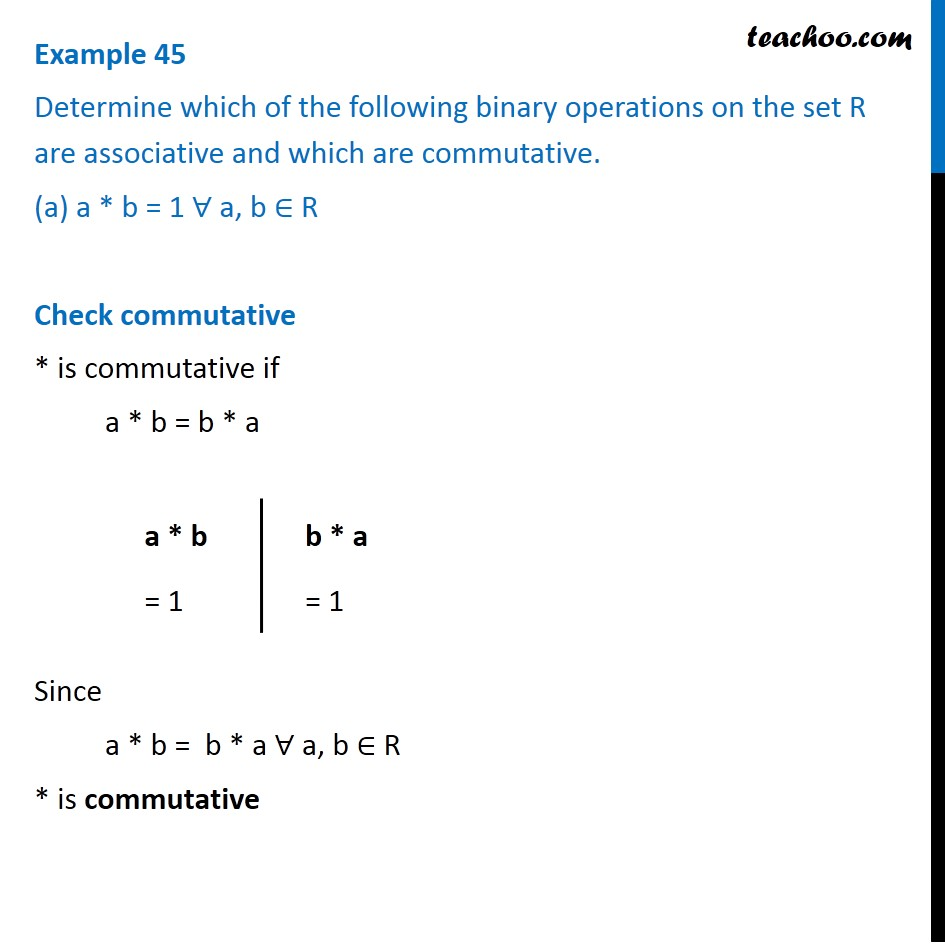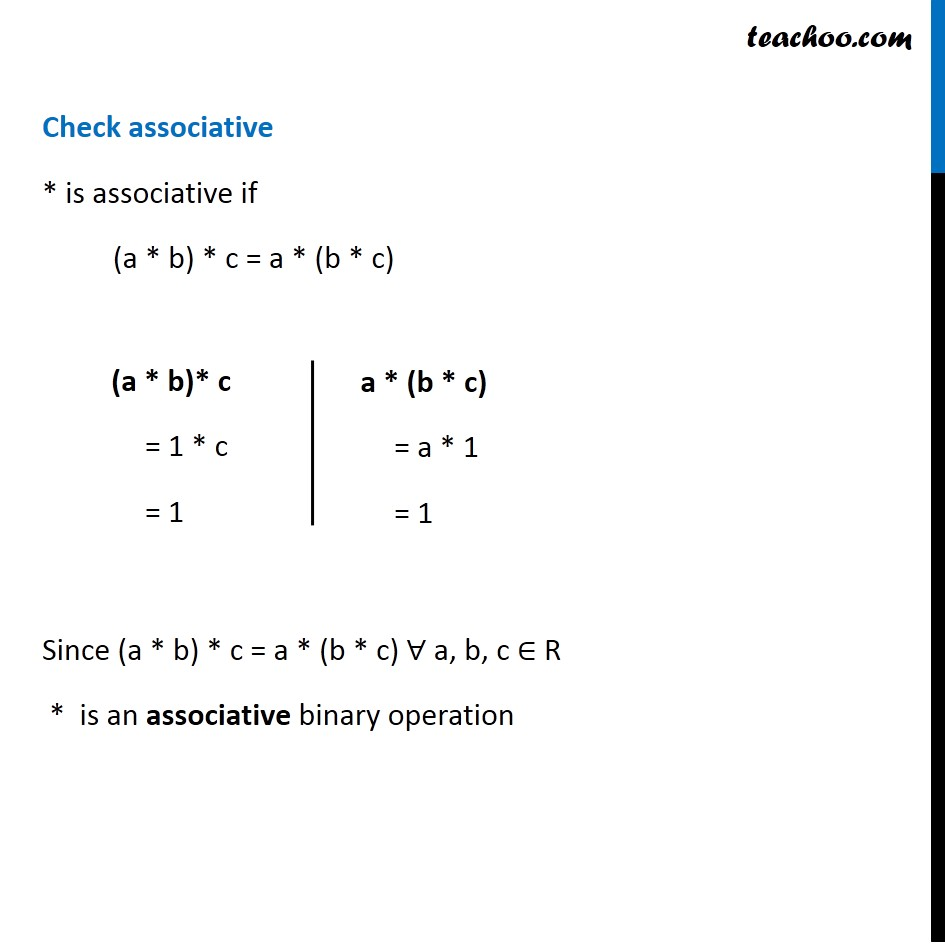Whether binary commutative/associative or not

Chapter 1 Class 12 Relation and Functions
Concept wiseIntroducing your new favourite teacher - Teachoo Black, at only ₹83 per month

### Transcript

Example 45 Determine which of the following binary operations on the set R are associative and which are commutative. (a) a * b = 1 ∀ a, b ∈ R Check commutative * is commutative if a * b = b * a Since a * b = b * a ∀ a, b ∈ R * is commutative a * b = 1 Check associative * is associative if a (a * b) * c = a * (b * c) Since (a * b) * c = a * (b * c) ∀ a, b, c ∈ R * is an associative binary operation Check associative * is associative if a (a * b) * c = a * (b * c) Since (a * b) * c = a * (b * c) ∀ a, b, c ∈ R * is an associative binary operation a * (b * c) = a * 1 = 1